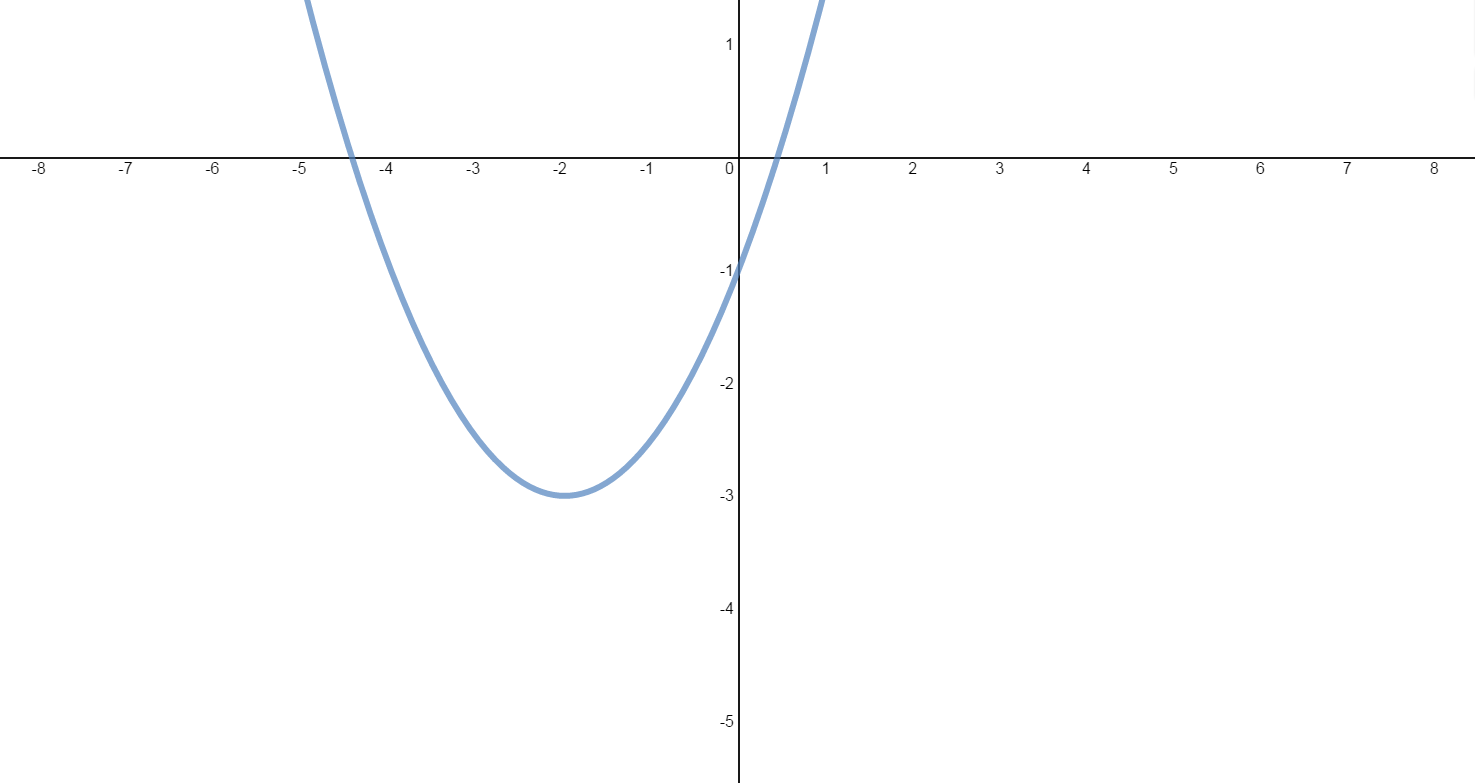# Slope and equation of tangent line

### Slope and equation of tangent line

The derivative of a function is interpreted as the slope of the tangent line to the curve of the function at a certain given point. In this section, we will explore the meaning of a derivative of a function, as well as learning how to find the slope-point form of the equation of a tangent line, as well as normal lines, to a curve at multiple given points.

#### Lessons

Point-Slope Form of a line with slope m through a point $(x_1,y_1): m=\frac{y-y_1}{x-x_1}$

Tangent Line & Normal Line
The normal line to a curve at a particular point is the line through that point and perpendicular to the tangent line.
• Introduction
Connecting: Derivative & Slope & Equation of Tangent Line
Exercise: The graph of the quadratic function $f\left( x \right) = \frac{1}{2}{x^2} + 2x - 1$ is shown below.a)
Find and interpret $f'\left( x \right)$.

b)
Find the slope of the tangent line at:
i) $x = - 1$
ii) $x = 2$
iii) $x = - 7$
iv) $x = - 4$
v) $x = - 2$

c)
Find an equation of the tangent line at:
i) $x = 2$
ii) $x = - 4$
iii) $x = - 2$

• 1.
Determining Equations of the Tangent Line and Normal Line
Consider the function: $f(x)=\frac{x}{32}(\sqrt{x}+{^3}\sqrt{x})$
a)
Determine an equation of the tangent line to the curve at $x=64$.

b)
Determine an equation of the normal line to the curve at $x=64$.

• 2.
Locating Horizontal Tangent Lines
a)
Find the points on the graph of $f(x)=2x^3-3x^2-12x+8$ where the tangent is horizontal.

b)
Find the vertex of each quadratic function:
$f(x)=2x^2-12x+10$
$g(x)=-3x^2-60x-50$

• 3.
Locating Tangent Lines Parallel to a Linear Function
Consider the Cubic function: $f(x)=x^3-3x^2+3x$
i) Find the points on the curve where the tangent lines are parallel to the line $12x-y-9=0$.
ii) Determine the equations of these tangent lines.

• 4.
Determining Lines Passing Through a Point and Tangent to a Function
Consider the quadratic function: $f(x)=x^2-x-2$
a)
Draw two lines through the point (3, -5) that are tangent to the parabola.

b)
Find the points where these tangent lines intersect the parabola.

c)
Determine the equations of both tangent lines.

• 5.
Locating Lines Simultaneously Tangent to 2 Curves
Consider the quadratic functions:
$f(x)=x^2$
$g(x)=\frac{1}{4}x^2+3$
a)
Sketch each parabola.

b)
Determine the lines that are tangent to both curves.